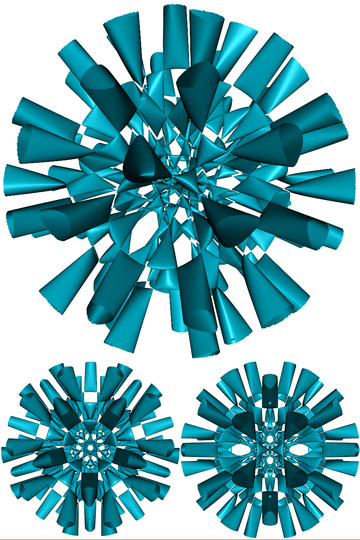#Journées de Géométrie Algébrique Poitiers-Bordeaux

24-25 November 2016
Poitiers
Europe/Paris timezone

## Degenerations of Nikulin surfaces and moduli of curves

25 Nov 2016, 11:20
50m
0-6 (Poitiers)

Board: 8
Exposé de 50 min

### Speaker

Prof. Andreas Knutsen

### Description

Nikulin surfaces are surfaces arising as quotiens of K3 surfaces by a symplectic involution. They have eight nodes (arising from the eight fixed points of the involution), and their desingularizations are smooth K3 surfaces with eight $(-2)$-curves whose sum is $2$-divisible in the Picard group. A particular feature is that their smooth hyperplane sections carry a nontrivial $2$-torsion element in their Picard group that is induced from a line bundle on the surface. There is therefore a natural moduli map from the space $P_g$ of pairs $(S,C)$ where $S$ is a Nikulin surface and $C$ is a smooth genus $g$ hyperplane section of it to the moduli space $R_g$ of genus $g$ Prym curves, that is, of pairs $(C,\eta)$, where $\eta$ is a nontrivial $2$-torsion element in $Pic(C)$. I will give an overview of recent results on this map obtained in a work in progress with Margherita Lelli-Chiesa and Alessandro Verra and how degenerations of Nikulin surfaces to surfaces that are birational to unions of rational ruled surfaces are of help.

### Presentation Materials

There are no materials yet.